Courses

# Lakhmir Singh & Manjit Kaur: Reflection of Light, Solutions- 3 Class 10 Notes | EduRev

## Class 10 : Lakhmir Singh & Manjit Kaur: Reflection of Light, Solutions- 3 Class 10 Notes | EduRev

The document Lakhmir Singh & Manjit Kaur: Reflection of Light, Solutions- 3 Class 10 Notes | EduRev is a part of the Class 10 Course Lakhmir Singh & Manjit Kaur Solutions: Class 10 Science.
All you need of Class 10 at this link: Class 10

Question 37: An object is 100 mm in front of a concave mirror which produces an upright image (erect image). The radius of curvature of the mirror is :
(a) less than 100 mm
(b) between 100 mm and 200 mm
(c) exactly 200 mm
(d) more than 200 mm

Solution : Since the image formed is upright, the object lies within the focus of the concave mirror.
So, f > 100 mm
We know R = 2f
So, R > 200 mm
i.e. Radius of curvature is more than 200 mm.

Question 38: A virtual, erect and magnified image of an object is to be produced with a concave mirror of focal length 12 cm. Which of the following object distance should be chosen for this purpose ?
(i) 10 cm
(ii) 15 cm
(iii) 20 cm

Solution : 10 cm, because if the object is placed between the pole and focus of the concave mirror only then the image formed is virtual, erect and magnified.

Question 39: A concave mirror has a focal length of 25 cm. At which of the following distance should a person hold his face from this concave mirror so that it may act as a shaving mirror ?
(a) 45 cm
(b) 20 cm
(c) 25 cm
(d) 30 cm

Solution : 20 cm, because if the object is placed between the pole and focus of the concave mirror only then the image formed is erect and magnified.

Question 40: An object is placed at the following distances from a concave mirror of focal length 15 cm, turn by turn :
(a) 35 cm
(b) 30 cm
(c) 20 cm
(d) 10 cm
Which position of the object will produce :
(i) a magnified real image ?
(ii) a magnified virtual image ?
(iii) a diminished real image ?
(iv) an image of same size as the object ?

Solution : (i) A magnified real image – When the object is placed at a distance of 20 cm from the mirror i.e., between focus and centre of curvature.
(ii) A magnified virtual image – When the object is placed at a distance of 10 cm from the mirror i.e., within its focus.
(iii) A diminished real image – When the object is placed at a distance of 35 cm from the mirror i.e. beyond the centre of curvature.
(iv) An image of same size as the object – When the object is placed at a distance of 30 cm from the mirror i.e., at the centre of curvature.

Page No:192

Question 1: According to the “New Cartesian Sign Convention” for mirrors, what sign has been given to the focal length of :
(i) a concave mirror ?
(ii) a convex mirror ?

Solution : (i) Negative
(ii) Positive

Question 2: Which type of mirror has :
(a) positive focal length ?
(b) negative focal length ?

Solution : (a) Convex mirror.
(b) Concave mirror.

Question 3: What is the nature of a mirror having a focal length of, +10 cm ?

Solution : Convex mirror (since focal length is positive).

Question 4: What kind of mirror can have a focal length of, – 20 cm ?

Solution : Concave mirror (since focal length is negative).

Question 5: Complete the following sentence :
All the distances are measured from the……….. of a spherical mirror.

Solution : Pole

Question 6: What sign (+ve or -ve) has been given to the following on the basis of Cartesian Sign Convention ?
(a) Height of a real image.
(b) Height of a virtual image.

Solution : (a) Negative.
(b) Positive.

Question 7: Describe the New Cartesian Sign Convention used in optics. Draw a labelled diagram to illustrate this sign convention.

Solution : According to the New Cartesian Sign Convention:
(i) All the distances are measured from pole of the mirror as origin.
(ii) Distances measured in the same direction as that of incident light are taken as positive.
(iii) Distances measured against the direction of incident light are taken as negative.
(iv) Distances measured upward and perpendicular to the principal axis are taken as positive.
(v) Distance measured downward and perpendicular to the principal axis are taken as negative.

Page No:193

Question 8: Giving reasons, state the ‘signs’ (positive or negative) which can be given to the following :
(a) object distance (u) for a concave mirror or convex mirror
(b) image distances (v) for a concave mirror
(c) image distances (v) for a convex mirror

Solution : (a) Object distance (u) for a concave mirror or convex mirror is always negative because an object is always placed to the left side of the mirror and the distances towards the left of the mirror are always negative.
(b) In case of a concave mirror, if the image is formed on the left side of the mirror, then the image distance (v) will be negative and if the image is formed on the right side of the mirror, then the image distance (v) will be positive. This is because distances measured to the left of the mirror are negative and to the right of the mirror is positive.
(c) Image distances (v) for a convex mirror is always positive because the image is always formed behind the mirror.

Page No:198

Question 1: If the magnification of a body of size 1 m is 2, what is the size of the image ?

Solution :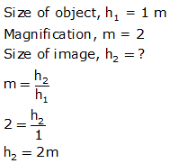Question 2: What is the position of the image when an object is placed at a distance of 20 cm from a concave mirror of focal length 20 cm ?

Solution : Object distance, u = -20 cm

Focal length. f = -20 cm (concave mirror)

Image d istance. v = ?

We know that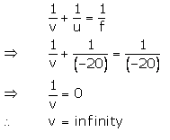Question 3: What is the nature of image formed by a concave mirror if the magnification produced by the mirror is (a) + 4, and (b) -2 ?

Solution : (a) If m = + 4. then the image is virtual and erect.
(b) If m = - 2. then the image is real and inverted.

Question 4: State the relation between object distance, image distance and focal length of a spherical mirror (concave mirror or convex mirror).

Solution :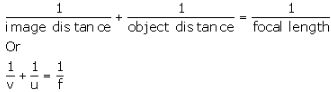v = distance of image from mirror
u = distance of object from mirror
f = focal length of mirror.

Question 5: Write the mirror formula. Give the meaning of each symbol which occurs in it.

Solution : Mirror formula is given belowv = distance of image from mirror
u = distance of object from mirror
f = focal length of mirror.

Question 6: What is the ratio of the height of an image to the height of an object known as ?

Solution : The ratio of height of an image to the height of an object is known as magnification.

Question 7: Define linear magnification produced by a mirror.

Solution : The ratio of the height of image to the height of object is known as linear magnification.

Question 8: Write down a formula for the magnification produced by a concave mirror.
(a) in terms of height of object and height of image
(b) in terms of object distance and image distance

Solution : Here = - 20 cm : f = - 10 cm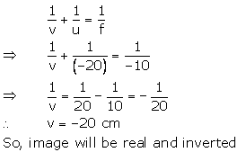Question 9: Describe the nature of image formed when the object is placed at a distance of 20 cm from a concave mirror of focal length 10 cm.

Solution : Here = - 20 cm : f = - 10 cmQuestion 10: Fill in the following blanks with suitable words :
(a) If the magnification has a plus sign, then image is…………. and……….
(b) If the magnification has a minus sign, then the image is……….. and……….

Solution : (a) virtual; erect
(b) real; inverted

Question 11: An object is placed at a distance of 10 cm from a concave mirror of focal length 20 cm.
(a) Draw a ray diagram for the formation of image.
(b) Calculate the image distance.
(c) State two characteristics of the image formed.

Solution :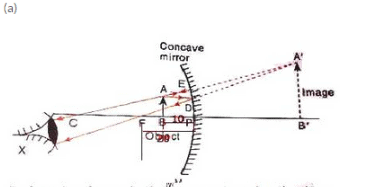fig. formation of image by the concave mirror when the object is palced between its pole and focus.

Here = - 20 cm : u = - 10 cm v = ?

We know that(c) Characteristics of image formed
(i) Image is virtual.
(ii) Image is erect.

Question 12: If an object of 10 cm height is placed at a distance of 36 cm from a concave mirror of focal length 12 cm, find the position, nature and height of the image.

Solution : h1 = 10cm. u = -36cm, f= -12cm

We know that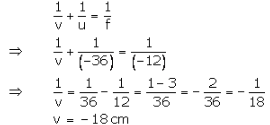∴ The position of the image is 18 cm in front of the mirror.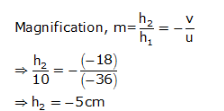The image formed is real and inverted.

Question 13: At what distance from a concave mirror of focal length 10 cm should an object 2 cm long be placed in order to get an erect image 6 cm tall ?

Solution : f=-10cm. h1 = 2cm. h2 = 6cm (erect image)
u = ?
We know that: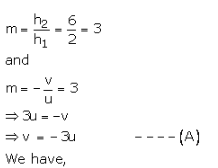The object should be placed at a distance of 6.66 cm on the left side of the mirror.

Question 14: When an object is placed at a distance of 15 cm from a concave mirror, its image is formed at 10 cm in front of the mirror. Calculate the focal length of the mirror.

Solution : u = -15 cm, v = -10cm
f = ?

We know that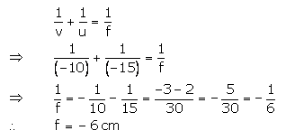Question 15: An object 3 cm high is placed at a distance of 8 cm from a concave mirror which produces a virtual image 4.5 cm high :
(i) What is the focal length of the mirror ?
(ii) What is the position of image ?
(iii) Draw a ray-diagram to show the formation of image.

Solution : h= 3cm. u = -8cm, h2 = 4.5 (virtual image)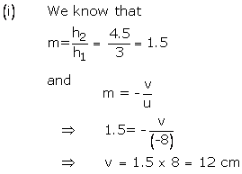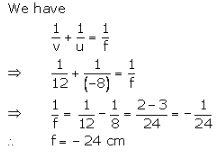(ii) v = 12cm

So, the image is formed 12 cm behind the concave mirror.

Question 16: A converging mirror forms a real image of height 4 cm of an object of height 1 cm placed 20 cm away from the mirror :
(i) Calculate the image distance.
(ii) What is the focal length of the mirror ?

Solution :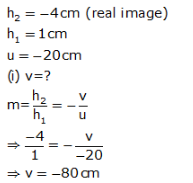Image forms in front of the concave mirror.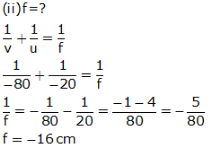Question 17: An object of size 7.0 cm is placed at 27 cm in front of a concave mirror of focal length 18 cm. At what distance from the mirror should a screen be placed so that a sharp focussed image can be obtained ? Find the size and nature of image.
[Hint. Find the value of image distance (v) first. The screen should be placed from the mirror at a distance equal to image distance].

Solution : Given : h1 = 7 cm, u = -27cm, f = -18cm,

We know that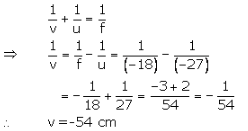The screen should be placed at a distance of 54 cm in front of the concave mirror.

And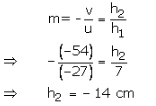Image is 14 cm in size, real and inverted.

Question 18: An object 3 cm high is placed at a distance of 10 cm in front of a converging mirror of focal length 20 cm. Find the position, nature and size of the image formed.

Solution : Given : h= 3cm, u = -10cm, f = -20cm,

We know that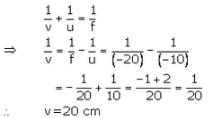The image is formed at a distance of 20 cm behind the mirror.

And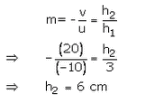Image is 6 cm in size , virtual and erect.

Question 19: A concave mirror has a focal length of 4 cm and an object 2 cm tall is placed 9 cm away from it. Find the nature, position and size of the image formed.

Solution : h1 = 2cm, u =-9cm, f = -4cm

We know that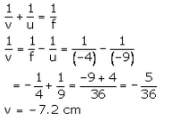The image is formed at a distance 7.2 cm in front of the mirror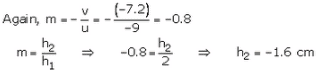So, image is 1.6 cm in size, real and inverted.

Question 20: When an object is placed 20 cm from a concave mirror, a real image magnified three times is formed. Find :
(a) the focal length of the mirror.
(b) Where must the object be placed to give a virtual image three times the height of the object ?

Solution : Given : u = -20cm.m = - 3, for the real image

(a) We know that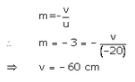We have,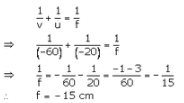(b) For virtual image m = 3, and f = -15crn

We know that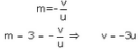We have,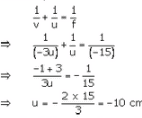So object should be placed 10cm from the concave mirror.

Question 21: A dentist’s mirror has a radius of curvature of 3 cm. How far must it be placed from a small dental cavity to give a virtual image of the cavity that is magnified five times ?

Solution : R = -3crn (concave mirror)
m = 5 (virtual image)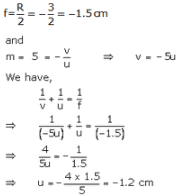The mirror should be placed 1.2cm away from the dental cavity.

Question 22: A large concave mirror has a radius of curvature of 1.5 m. A person stands 10 m in front of the mirror. Where is the person’s image ?

Solution : R = -1.5crn (concave mirror)
u = -10 cm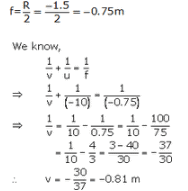The perscn's image will be 0.81 m in front of ccncave mirror.

Question 23: An object of 5.0 cm size is placed at a distance of 20.0 cm from a converging mirror of focal length 15.0 cm. At what distance from the mirror should a screen be placed to get the sharp image ? Also calculate the size of the image.

Solution : h1= 5.0cm. u = -20cm. f = -15cm. v=?. h2=?

We know that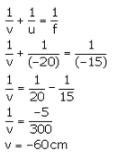The screen should be placed 60cm in front of the mirror.

And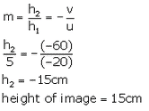Question 24: A concave mirror produces three times enlarged virtual image of an object placed at 10 cm in front of it. Calculate the radius of curvature of the mirror.

Solution : m = 3 (virtual image)
u =-10cm
R = ?

We know that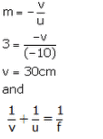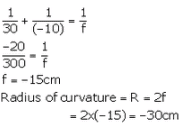Question 25: A bright object 50 mm high stands on the axis of a concave mirror of focal length 100 mm and at a distance of 300 mm from the concave mirror. How big will the image be ?

Solution : h1 = 50mm. f = -100mm. u = -300mm. h1=?

We have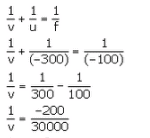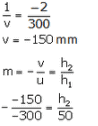The Image will be 25 mm high.

Question 26: How far should an object be placed from the pole of a converging mirror of focal length 20 cm to form a real image of the size exactly 1/4th the size of the object ?

Solution : f = -20cm, m = -1/4 (real image)

We know that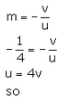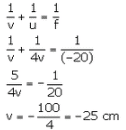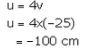The object should be placed 100 cm to the left of the mirror.

Question 27: When an object is placed at a distance of 50 cm from a concave spherical mirror, the magnification produced is, -1/2 Where should the object be placed to get a magnification of, -1/5?

Solution :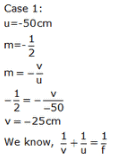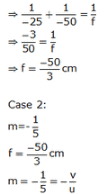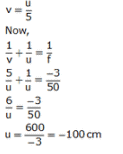Question 28: An object is placed (a) 20 cm, (b) 4 cm, in front of a concave mirror of focal length 12 cm. Find the nature and position of the image formed in each case.

Solution :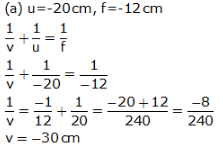The image is formed at a distance of 30 cm in front of the mirror. The image is real and inverted.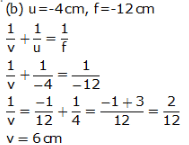The image is formed at a distance of 6 cm behind the mirror. The image is virtual and erect.

Question 29: A concave mirror produces a real image 1 cm tall of an object 2.5 mm tall placed 5 cm from the mirror. Find the position of the image and the focal length of the mirror.

Solution : h2 = 1cm = 10mm (real image), h1 = 2.5mm. u = -5cm = -50mm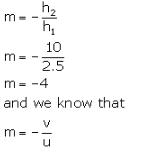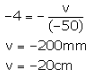The image is formed 20 cm in front of the mirror.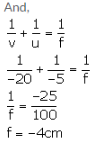Question 30: A man holds a spherical shaving mirror of radius of curvature 60 cm, and focal length 30 cm, at a distance of 15 cm, from his nose. Find the position of image, and calculate the magnification.

Solution :

Radius of curvature, R = -60cm (concave mirror)
f = -30cm. u = -15cm

We have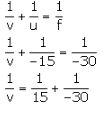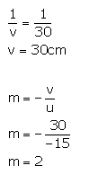So. the the image is formed 30 cm behind the mirror and the magnification is +2.

Question 31: (a) An object is placed just outside the principal focus of concave mirror. Draw a ray diagram to show how the image is formed, and describe its size, position and nature.
(b) If the object is moved further away from the mirror, what changes are there in the position and size of the image ?
(c) An object is 24 cm away from a concave mirror and its image is 16 cm from the mirror. Find the focal length and radius of curvature of the mirror, and the magnification of the image.

Solution :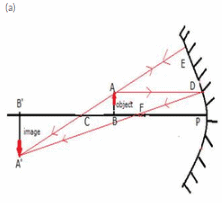The image is real inverted and magnified It is formed beyond the centre of curvature of the mirror.
(b) If the object is moved further away from the mirror. the image is tamed nearer to the mirror and its size goes on decreasing.
(c) u = -24cm
v = -1.6cm
f = ?.R = ?.m = ?
weknow that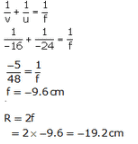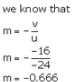Offer running on EduRev: Apply code STAYHOME200 to get INR 200 off on our premium plan EduRev Infinity!

61 docs

,

,

,

,

,

,

,

,

,

,

,

,

,

,

,

,

,

,

,

,

,

,

,

,

;